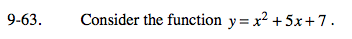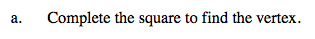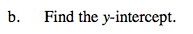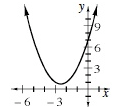Home > A2C > Chapter 9 > Lesson 9.1.3 > Problem9-63

9-63.
1. Consider the function y = x2 + 5x + 7. Homework Help ✎

1. Complete the square to find the vertex.

2. Find the y-intercept.

3. Use the vertex, the y-intercept, and the symmetry of parabolas to find a third point and sketch the graph.$\text{Vertex: }\left(-\frac{5}{2},\,\frac{3}{4}\right)$(0, 7)